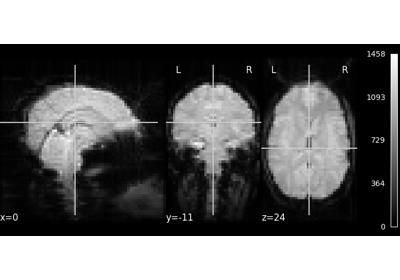Note

This page is a reference documentation. It only explains the function signature, and not how to use it. Please refer to the user guide for the big picture.

Extract signals from images using specified mask.

Read the time series from the given Niimg-like object, using the mask.

Parameters
imgs: list of 4D Niimg-like objects

See http://nilearn.github.io/manipulating_images/input_output.html Images to be masked. list of lists of 3D images are also accepted.

See http://nilearn.github.io/manipulating_images/input_output.html 3D mask array: True where a voxel should be used.

dtype: numpy dtype or ‘f’

The dtype of the output, if ‘f’, any float output is acceptable and if the data is stored on the disk as floats the data type will not be changed.

smoothing_fwhm: float

(optional) Gives the size of the spatial smoothing to apply to the signal, in voxels. Implies ensure_finite=True.

ensure_finite: bool

If ensure_finite is True (default), the non-finite values (NaNs and infs) found in the images will be replaced by zeros.

Returns
session_series: numpy.ndarray

2D array of series with shape (image number, voxel number)

Notes

When using smoothing, ensure_finite is set to True, as non-finite values would spread across the image.

## 8.7.8.1. Examples using `nilearn.masking.apply_mask`¶